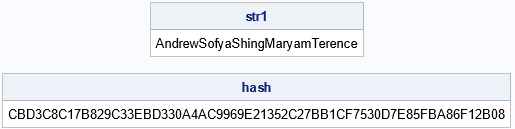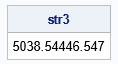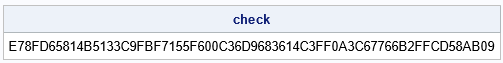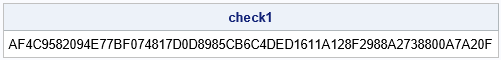Have you ever typed your credit card into an online order form and been told that you entered the wrong number? Perhaps you wondered, "How do they know that the numbers I typed do not make a valid credit card number?" The answer is that credit card numbers and other account numbers incorporate a simple rule that protects against many typographical errors. In general, a rule that validates a block of data is called a checksum. The checksum that is used by credit cards is computed by using the Luhn algorithm or the "mod 10" algorithm. Chris Hemedinger wrote a blog post that shows how to implement the Luhn checksum algorithm in SAS.

You can also use checksum methods to ensure that information or data is not changed after it is created. I recently needed to ensure that some data was not being corrupted as it was passed along a complicated SAS/IML program. I was able to use the SHA256 function in SAS to create a checksum for the data. Chris Hemedinger has a blog post that describes the SHA256 function and how to call it from the SAS DATA step to ensure the data integrity of character data. Although he doesn't say it, you can use the SHA256 function on numerical data by applying a format to the value (for example, by using the PUTN function in SAS). The format will determine the value of the checksum.

This article shows how to use the SHA256 function to create a checksum for character and numerical data. For SAS/IML programmers, I also show how to use a checksum to ensure that items in a SAS/IML list have not changed since the list was created.

### Hashing character values

You can use any hash function to create a checksum. SAS supports a large variety of hash functions. This article uses the SHA256 function, but you can use a different function if you prefer.

The following example illustrates how you can use a hash function for an array of character values. In the SAS/IML language, the ROWVEC function takes a matrix of input values and converts them into a one-dimensional row vector. The ROWCATC function takes a character matrix and concatenates the values across columns, removing all spaces. Thus, the SAS/IML expression rowcatc(rowvec(m)) returns a string that concatenates the elements in m. You can perform a similar operation in the SAS DATA step by using the CATS function.

```/* a simple checksum: SHA256 applied to data strings */ proc iml; ID = {"Andrew", "Sofya", "Shing", "Maryam", "Terence"};   str1 = rowcatc(rowvec(ID)); hash = sha256(str1); print str1, hash[F=\$hex64.];```The program takes the names of five people, concatenates them together into a long string, and then applies the SHA256 hash function. The output is a fixed-length string. For the SHA256 function, the output string contains 256 bits. Since each hexadecimal digit can represent four bits of information, you can display this 256-bit value by using a 64-character hexadecimal string. This is one of the advantages of using a hash function: regardless of the length of the original string, the output is a string of a known length.

### Hashing numerical values

If you want to hash an array of character values, you can use the PUTN function in SAS to apply a format, which converts the array of numbers into a string. This article uses the BEST12. format. If you use a different format, you will get a different string and a different hash value.

In SAS 9.4, character strings are limited to 32,767 characters. (In SAS Viya, you can use the VARCHAR type to store strings of any length.) If you allocate 12 characters to each number, you can encode at most 32767/12 = 2730 numbers into a single string. There are ways to get around this, but for simplicity, if an array contains more than 100 values, I will use only the first 100 values to create the hash value. This is implemented in the following SAS/IML user-defined function, which takes a matrix of numbers as input and returns a long string representation of the numbers:

```start NumToStr(m, maxN=100); if nrow(m)*ncol(m) < maxN then s = putn(m, "BEST12."); /* apply format to all values */ else s = putn(m[1:maxN], "BEST12."); /* apply format to first 100 values */ return( rowcatc(rowvec(s)) ); /* concatenate values into one string */ finish;   Height = {175, 143, 165, 157, 161}; /* cm */ Weight = {50, 38.5, 44, 46.5, 47}; /* kg */ corr = 0.8805;   str2 = NumToStr(Height); str3 = NumToStr(Weight); str4 = NumToStr(corr); print str3;```The function converts an array of numbers into a string of formatted values. The function is tested by using two arrays and one scalar value. One of the strings is shown. You can see that the formatted Weight values (which include decimal points) are concatenated into a string.

### Creating a checksum

The previous sections created four strings: str1, str2, str3, and str4. Notice that the correlation value (0.8972) is dependent on the Height and Weight data values. If the values in those arrays were to change (either accidentally or intentionally), it would be useful to know that the correlation value is no longer valid. One way to find out if any information has changed is to create a checksum for the original information and periodically compare the original checksum to the checksum for the current state of the information. The following statements compute the checksum for the original data by concatenating the strings for the ID, Height, Weight, and Corr variables and computing the SHA256 value of the result:

```s = cats(str1, str2, str3, str4); check = sha256(s); print check[F=\$hex64.];```The output is a checksum. If you modify any of the numbers or strings in these arrays, then (with high probability) the new checksum will not match the original checksum. For example, the following statements compute the checksum for the correlation equal to "0.89". The new checksum is different from the original checksum, which tells you that one or more data values have changed.

```/* What if the numbers or order of numbers change? */ s = cats(str1, str2, str3, "0.89"); check1 = sha256(s); print check1[F=\$hex64.];```### A checksum for a SAS/IML list

This section shows one way to automate the computations in the previous sections. In the SAS/IML Language, it is often convenient to use a list to pack related information into a single object that you can pass to functions. This section shows how to compute the checksum for a list of arbitrary items.

The program defines the following functions:

• The CharToStr function concatenates up to 100 elements in a character matrix. The result is a single string.
• The NumToStr function applies the BEST12. format to up to 100 elements in a numeric matrix. These formatted values are concatenated together to form a single string.
• The GetChecksum function iterates over the items in a list. If an item is a character matrix, call the CharToStr function. If the item is a numeric matrix, call the NumToStr function. For simplicity, other items are skipped in this implementation. The strings for each item are concatenated together. The SHA256 function creates a hash value for the concatenated strings.

This process is demonstrated by using the same data as in the previous sections:

```proc iml; start CharToStr(s, maxN=100); if nrow(s)*ncol(s) < maxN then return( rowcatc(rowvec(s)) ); /* concatenate all values */ else return( rowcatc(rowvec(s[1:maxN])) ); /* concatenate 100 values */ finish;   start NumToStr(m, maxN=100); if nrow(m)*ncol(m) < maxN then s = putn(m, "BEST12."); /* apply format to all values */ else s = putn(m[1:maxN], "BEST12."); /* apply format to first 100 values */ return( CharToStr(s) ); /* concatenate values into one string */ finish;   /* get hash of the numerical and character elements of a list*/ start GetChecksum(L); allStr = ""; do i = 1 to ListLen(L); m = L\$i; if type(m)='N' then str = NumToStr(m); else if type(m)='C' then str = CharToStr(m); else str = ""; /* you could handle other types here */ allStr = cats(allStr, str); end; checksum = sha256(allStr); return checksum; finish;   ID = {"Andrew", "Sofya", "Shing", "Maryam", "Terence"}; Height = {175, 143, 165, 157, 161}; /* cm */ Weight = {50, 38.5, 44, 46.5, 47}; /* kg */ corr = 0.8805;   L = [ID, Height, Weight, corr]; /* list with four items */ check = GetChecksum(L); print check[F=\$hex64.];```The hash value in this program is identical to the hash value for the original data. That is because the items were packed into the list in the same order.

This process was motivated by a real-life problem. I was writing a complicated program that pre-computes certain statistics and then calls a series of functions. I wanted to ensure that the items in the list were not modified as the list was passed from function to function. One way to do that is to compute the checksum of the original items in the list and compare it to the checksum at the end of the program.

### Summary

This article discusses how to use a hash function to compute a checksum to ensure data integrity. The article uses the SHA256 hash function, but SAS supports other hash functions. A hash function takes an arbitrary string as input and returns a fixed-length string, called the hash value. With high probability, the hash value "identifies" the data: if you change the data, you are likely to obtain a different hash value. You can use this fact to compute a checksum for the original data and compare it to the checksum for the data at a later time. If the checksum has changed, the data have been corrupted.

Share